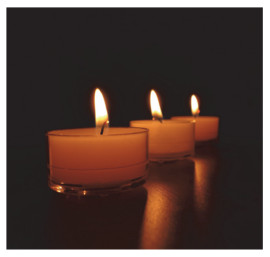## A Travel of the Primitive Tribe (Part 1)

FANG Simin, LAN Quan, LIU Wei, FENG Hongyan, WANG Honglei, GUO Linhua, WU Hong, ZHU Pingping,

 基金资助: 中国科学院2018年科普教育类项目.  2018D0038

 Fund supported: 中国科学院2018年科普教育类项目.  2018D0038Abstract

Chinese culture is like a vast starry sky where poetry and idioms are the bright pearls. This article followed the steps of Dahua and Xiaoxue into a primitive tribe, where they explored the charm of chemistry in poetry and idioms and appreciated the profoundness of Chinese culture.

Keywords： Poetry ; Idiom ; Chemistry

FANG Simin. A Travel of the Primitive Tribe (Part 1). University Chemistry[J], 2019, 34(8): 68-71 doi:10.3866/PKU.DXHX201904037

“大华，大华，快起来啦！”小雪一早大声喊道，“要迟到了！”

“今天是周末，怎么会迟到。”大华不满地翻了个身，想要继续他的美梦。

### 图1### 图2${\rm{2}}{{\rm{C}}_{{\rm{22}}}}{{\rm{H}}_{{\rm{46}}}} + {\rm{ }}67{{\rm{O}}_2}\mathop = \limits^{{\rm{点燃}}} 44{\rm{C}}{{\rm{O}}_2} \uparrow {\rm{ }} + {\rm{ }}46{{\rm{H}}_{\rm{2}}}{\rm{O}}$
${\rm{2}}{{\rm{C}}_{{\rm{28}}}}{{\rm{H}}_{{\rm{58}}}} + {\rm{ }}85{{\rm{O}}_2}\mathop = \limits^{{\rm{点燃}}} 56{\rm{C}}{{\rm{O}}_2} \uparrow {\rm{ }} + {\rm{ }}58{{\rm{H}}_{\rm{2}}}{\rm{O}}$

### 图3$\begin{array}{*{20}{c}}{{\rm{CaC}}{{\rm{O}}_3} + {\rm{C}}{{\rm{O}}_2} + {{\rm{H}}_2}{\rm{O}} = {\rm{Ca}}{{\left({{\rm{HC}}{{\rm{O}}_3}} \right)}_2}}\\{{\rm{Ca}}{{\left({{\rm{HC}}{{\rm{O}}_3}} \right)}_2} = {\rm{CaC}}{{\rm{O}}_3} \downarrow + {\rm{C}}{{\rm{O}}_2} \uparrow + {{\rm{H}}_2}{\rm{O}}}\end{array}$

### 图4$\begin{array}{l}3{{\rm{O}}_{\rm{2}}}\mathop = \limits^{{\rm{放电}}} 2{{\rm{O}}_{\rm{3}}}\\{{\rm{O}}_3} = {{\rm{O}}_2} + {\rm{O}}\end{array}$

## 参考文献 原文顺序 文献年度倒序 文中引用次数倒序 被引期刊影响因子

/

 〈〉